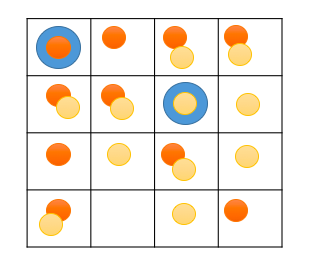According to wikipedia,

Backtracking is general algorithm for finding all (or some) solutions to some computational problems, notably constraint satisfaction problems, that incrementally builds candidates to the solutions, and abandons a candidate (backtracks) as soon as it determines that the candidate cannot possibly be completed to a valid solution.

I have compared backtracking to DFS in a previous post, so here I’ll just focus on the classic problems solved by backtracking.

## I. Permutation

46. Permutations: return all possible permutations given a list of distinct numbers.

This is a simple but classic problem. It has many different solutions, not limited to the ones I provide below. And the ideas of the solutions can be applied to many other problems.

Solution 1: simulate the way that we create permutations.
Suppose there are {1, 2, 3}, we can use all these numbers for Position 0; if we pick 1, then Poisition 1 is left with {2, 3}; if we pick 3 for Position 1, then we leave Position 2 with 2; so we have one permutation {1, 3, 2}. And then we backtrack, repeat the above process with different selections, and we’ll get another permutation.

Solution 2: in-place swap.

Solution 3: another implementation of in-place swap.

Solutioin 2 vs. Solution 3:

• The permutations generated by Solution 2 are not in lexicographical order; but the ones generated by Solution 3 are in lexicogrphical order
• For Solution 3, the numbers being swapped are adjacent in the original array

What causes the differences is that Solution 3 does not recover the order of numbers[i] and numbers[j] by using swap again and it makes a copy of the array so that the changes do not affect the array at the upper level.

Here is the process of applying Solution 2:

i j numbers output
0 0 1, 2, 3
1 1 1, 2, 3 x
1 2 1, 3, 2 x
0 1 2, 1, 3
1 1 2, 1, 3 x
1 2 2, 3, 1 x
0 2 3, 2, 1
1 1 3, 2, 1 x
1 2 3, 1, 2 x

Here is the process of applying Solution 3:

i j numbers output
0 0 1, 2, 3
1 1 1, 2, 3 x
1 2 1, 3, 2 x
0 1 2, 1, 3
1 1 2, 1, 3 x
1 2 2, 3, 1 x
0 2 3, 1, 2
1 1 3, 1, 2 x
1 2 3, 2, 1 x

When i = 0 and j = 2, Solution 2 does the swap based on {1, 2, 3}, but Solution 3 is based on the result of swapping numbers and numbers, that is, {2, 1, 3}, and that makes all the differences.

Note that the time complexity for the solutions above is O(n!).

Follow-up: 47. Permutations II, the list of numbers might contain duplicates, but the permutations are required to be unique.

It is natural to figure out a solution to make use of Solution 3 that we can compare the two numbers before swap them, if they are equal, we will just skip the swap and move the the next number, so what we need to do is to sort the array to make sure that the duplicates are adjacent.

## II. N-Queen

51. N-Queens: place n queens on an *nxn chessboard such that no two queens attack each other.

The idea is quite straightforward: place a queen on each row and try one row after another, if it does not work, backtrack to the last row and try another column. The key is to mark down which cells cannot be used when a queen is placed on the chessboard and unmark them when it is removed from the chessboard, as shown in the figure below.The time complexity is O(n!).

## III. Sudoku

37. Sudoku Solver: solve a Suduku puzzle.

The solution of sudoku is similar to the one of the N-queen problem, but a bit complicated. There are only two possibilites for each cell in the N-queen problem: a queen is placed in it or not; but for Sudoku, there are 9 possibilities, so we need to maintain the state of these 9 possibilities for each cell. When a number is filled in an empty cell, we need to update state for cells on the same row or on the same column or in the same block.
The time complexity is O(9^n).

## IV. More

17. Letter Combinations of a Phone Number: the Solution 1 of permutation can be directly applied to the problem.

22. Generate Parentheses: given n pairs of parentheses, write a function to generate all combinations of well-formed parentheses.

The time complexity is O(2^2n).